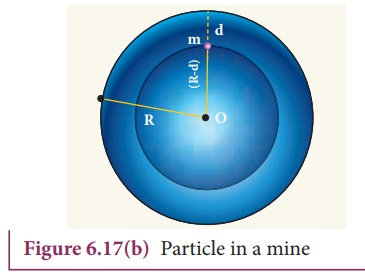Home | | Physics 11th std | Variation of g(acceleration of gravity) with altitude, depth and latitude

# Variation of g(acceleration of gravity) with altitude, depth and latitude

Consider an object of mass m at a height h from the surface of the Earth.

Variation of g with altitude, depth and latitude

Consider an object of mass m at a height h from the surface of the Earth. Acceleration experienced by the object due to Earth isIf h << Re

We can use Binomial expansion. Taking the terms upto first orderWe find that g’< . This means that as altitude h increases the acceleration due to gravity g decreases.

### EXAMPLE 6.7

1. Calculate the value of g in the following two cases:

a) If a mango of mass ½ kg falls from a tree from a height of 15 meters, what is the acceleration due to gravity when it begins to fall?

### Solutionb) Consider a satellite orbiting the Earth in a circular orbit of radius 1600 km above the surface of the Earth. What is the acceleration experienced by the satellite due to Earth’s gravitational force?

### SolutionThe above two examples show that the acceleration due to gravity is a constant near the surface of the Earth.

## Variation of g with depth:

Consider a particle of mass m which is in a deep mine on the Earth. (Example: coal mines in Neyveli). Assume the depth of the mine as d. To calculate g at a depth d, consider the following points.The part of the Earth which is above the radius (Re  d) do not contribute to the acceleration. The result is proved earlier and is given asHere M ’  is the mass of the Earth of radius (Re-d)

Assuming the density of Earth ρ to be constant,where M is the mass of the Earth and V its volume, Thus,ThusHere also  g ′ < g . As depth increases, g′ decreases. It is very interesting to know that acceleration due to gravity is maximum on the surface of the Earth but decreases when we go either upward or downward.

## Variation of g with latitude:

Whenever we analyze the motion of objects in rotating frames [explained in chapter 3] we must take into account the centrifugal force. Even though we treat the Earth as an inertial frame, it is not exactly correct because the Earth spins about its own axis. So when an object is on the surface of the Earth, it experiences a centrifugal force that depends on the latitude of the object on Earth. If the Earth were not spinning, the force on the object would have been mg. However, the object experiences an additional centrifugal force due to spinning of the Earth.This centrifugal force is given by mω2R .where λ is the latitude. The component of centrifugal acceleration experienced by the object in the direction opposite to g isTherefore,From the expression (6.52), we can infer that at equator, λ = 0; g’= g - ω2R. The acceleration due to gravity is minimum. At poles λ= 90; g’=g, it is maximum. At the equator, g’ is minimum.

### EXAMPLE 6.8

Find out the value of g in your school laboratory?

### Solution

Calculate the latitude of the city or village where the school is located. The information is available in Google search. For example, the latitude of Chennai is approximately 13 degree.

′ = g −ω 2 R cos2 λ

Here ω2R = (2x3.14/86400)2 x (6400x103) = 3.4x10−2 m s−2.

It is to be noted that the value of λ should be in radian and not in degree. 13 degree is equivalent to 0.2268 rad.

g′ = 9.8  ( 3.4 × 102 × ( cos 0.2268)2

g = 9.7677 m s−2

Study Material, Lecturing Notes, Assignment, Reference, Wiki description explanation, brief detail
11th Physics : Gravitation : Variation of g(acceleration of gravity) with altitude, depth and latitude |

Related Topics